Lakhmir Singh & Manjit Kaur: Sound, Solutions- 1

# Lakhmir Singh & Manjit Kaur: Sound, Solutions- 1 - Science Class 9

Page No:185

Solution 1
Yes, sound can travel through iron and as well as water.
Solution 2
No, sound cannot travel through vacuum.
Solution 3
Radio waves are used to communicate with one another on moon.
Solution 4
Solid – table
Liquid – water
Gas – air
Solution 5
Vacuum
Solution 6
Frequency
Solution 7
SI unit of frequency is hertz.
Solution 8
(a)Longitudinal wave
(b)Transverse wave
Solution 9
Speed of sound is more in steel (solid medium) as compared to water (liquid medium).
Solution 10
Sound travels faster in iron (being a solid medium).
Solution 11
Sound travels fastest in steel (solid medium).
Solution 12
(a)Sound travels slowest in gases.
(b)Sound travels fastest in solids.
Solution 13
(a) Speed of sound in copper = 3750m/s
(b) Speed of sound in aluminium = 5100m/s
Solution 14
It is more convenient to put the ear to the track to hear a train approaching from far away because sound travels faster in solids than in air.
Solution 15
Speed of sound (at 20oC) in:
(a) Air = 344 m/s
(b) Water =1498 m/s
(c) Iron =5130 m/s
Solution 16
Supersonic aircrafts
Solution 17
Supersonic Speed
Solution 18
Supersonic speed refers to the speed of an object which is greater than the speed of sound.
Solution 19
It is common observation that in the rainy season, the flash of lightning is seen first and the sound of thunder is heard a little later. That’s because, speed of
light is very high as compared to speed of sound in air.
Solution 20
Transverse and Longitudinal waves.
Solution 21
Transverse (water) waves.
Solution 22
Longitudinal (sound) waves.
Solution 23
(a) Longitudinal waves
(b) Transverse waves.
Solution 24
(a) Longitudinal waves
(b) Transverse waves
Solution 25
Transverse waves
Solution 26
An object should vibrate in order to produce sound.
Solution 27
Vocal cords vibrate in our voice box when we talk.
Solution 28
Tuning fork is used to produce sound in laboratory experiments.
Solution 29
The sound waves in air are longitudinal waves.
Solution 30
The conclusion from the observation is that the prongs of tuning fork are vibrating, and the vibrating prongs carry energy which gets transmitted to surrounding medium.

Page No - 186

Solution 31
False
Solution 32
Slowest: Sound
Fastest: light
Solution 33
Supersonic is used to denote a speed greater than the speed of sound.
Solution 34
Sound travels faster in hydrogen ( speed of sound in hydrogen is 1284m/s)
Solution 35
The number 256 on tuning fork signifies the frequency of tuning fork.
Solution 36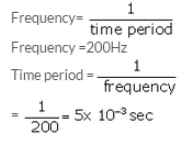Solution 37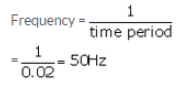Solution 38
Velocity of sound = Frequency x wavelength
Speed of sound in air is constant.
Hence, frequency x wavelength = constant
If frequency is doubled, wavelength is reduced to half.
Solution 39
The frequency in hertz is equal to the number of waves produced per second. In this case, 20 waves are produced per second, so the frequency of sound waves is 20 hertz.
Solution 40
(a) Vibrations
(b)Compressions; lower; rarefactions
(c)Hertz; wavelength; metres
(d) Vacuum
(e)Greater
(f) Decreases
Solution 41
Vacuum means empty space, region with no matter particles. Sound cannot travel through vacuum because vacuum has no molecules which can vibrate and carry sound waves.
Solution 42
The maximum displacement of the particles of the medium from their original undisturbed positions, when a wave passes through the medium, is called amplitude (A) of the wave.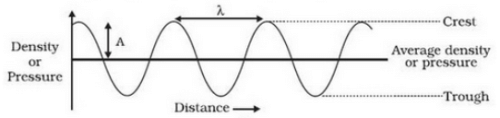Solution 43
(a) A wave in which the particles of the medium vibrate back and forth in the ‘same direction’, in which the wave is moving, is called a longitudinal wave. These
waves can be produced in all the three media: solids, liquids and gases. A wave in which the particles of the medium vibrate up and down, ‘at right angles’
to the direction in which the wave is moving, is called a transverse wave. It can be produced in solids and liquids but not in gases.
(b) Sound is a longitudinal wave.
Solution 44
Due to the very high speed of light we see the ball hitting the bat first. And it is due to comparatively lower speed of sound that the sound of hitting is heard a little later.
Solution 45
Light travels much faster than sound. Due to this, the flash of lightning is seen first and the sound of thunder is heard a little later.
Solution 46
Light travels much faster than sound. Due to this, the flash of gun shot is seen first and the sound of gun shot is heard a little later.
Solution 47
Sound waves in air: Longitudinal, Compression, Rarefaction
Water waves: Transverse, Crest, Trough
Solution 48
(a) Sound can be produced by the following methods:
(i) By vibrating strings (as in a sitar),
(ii) By vibrating air (as in a flute),
(iii) By vibrating membranes (as in a drum)
(iv) By vibrating plates (as in cymbals)
(b) Speed of sound wave= frequency x wavelength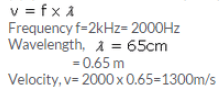Solution 49
This is due to the fact that when the ringing bell is held tightly with our hand, it stops vibrating and the sound coming from it also stops.
Solution 50
Sound is produced by the following objects:
(i) Vibrating stretched strings of sitar
(ii) Vibrating stretched membranes of tabla
(iii) Vibrating prongs of a tuning fork
(iv) Vibrating wings of mosquito
(v) Vibrating air columns in flute.
Solution 51
In most of the cases, a sound producing object vibrates so fast that we cannot see its vibrations with our eyes. The time inetrval between two successive vibration is lower than the persistence of vision. Hence we see the object in static state and not in vibration mode.
Solution 52
Fill water in a beaker up to its brim. Touch the surface of water with the prongs of a sound making tuning fork (which has been struck on a hard rubber pad). The prongs of tuning fork producing sound splash water. This shows that the prongs of a sound producing tuning fork are vibrating (moving forwards and backwards rapidly).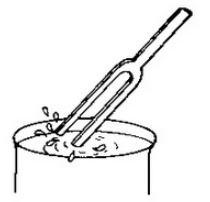`the prongs of a sound producing tuning fork splash water, so they are vibrating`

Solution 53
The sound of a gas travels through the vibrations of air layers so it reaches first, but the smell of gas reaches the person through the actual movement of the air
layers, which takes more time.
Solution 54
Frequency is number of vibrations produced per second i.e. 128 Hz.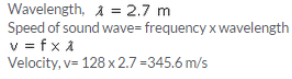Solution 55
Velocity of wave= 340m/s
Frequency= 512 Hz
Wavelength=?
Speed of sound wave=
frequency x wavelength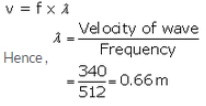Solution 56
The number of complete waves (or cycles) produced in one second is called frequency of the wave.
The time required to produce one complete wave (or cycle) is called time-period of the wave.
The time taken to complete one vibration is called time-period.
Relation between time-period and frequency of a wave is: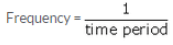Solution 57
A ringing bell suspended in a vacuum chamber cannot be heard outside because sound cannot travel through vacuum as it has no molecules which can vibrate and carry
sound waves.
Solution 58
Frequency, f=1020Hz
Velocity, v=340m/s
Wavelength =?
Speed of sound wave= frequency x wavelength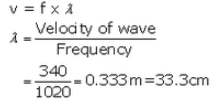Solution 59
A compression is that part of a longitudinal wave in which the particles of the medium are closer to one another than they normally are, and there is a momentary reduction in volume of the medium. It is a region of high pressure.
A rarefaction is that part of a longitudinal wave in which the particles of the medium are farther apart than normal, and there is a momentary increase in the volume of the medium. It is a region of low pressure.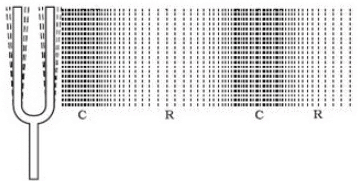The document Lakhmir Singh & Manjit Kaur: Sound, Solutions- 1 | Science Class 9 is a part of the Class 9 Course Science Class 9.
All you need of Class 9 at this link: Class 9

## Science Class 9

66 videos|355 docs|97 tests

## FAQs on Lakhmir Singh & Manjit Kaur: Sound, Solutions- 1 - Science Class 9

The underlying connection was closed: An unexpected error occurred on a receive.

## Science Class 9

66 videos|355 docs|97 testsExplore Courses for Class 9 examSignup to see your scores go up within 7 days! Learn & Practice with 1000+ FREE Notes, Videos & Tests.
10M+ students study on EduRev
Track your progress, build streaks, highlight & save important lessons and more!
Related Searches

,

,

,

,

,

,

,

,

,

,

,

,

,

,

,

,

,

,

,

,

,

,

,

,

;